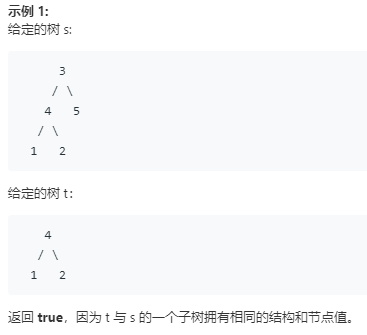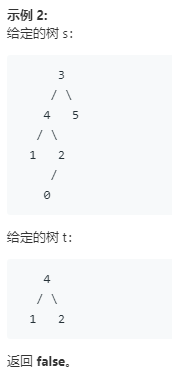# Leetcode 572：另一个树的子树

## 示例isSubtree()函数

s为空，则一定false；

isSub()函数

## 代码实现

bool isSub(TreeNode* s, TreeNode* t){ if (!s && !t) return true; if (!s || !t) return false; if (s->val != t->val) return false; return isSub(s->left, t->left) && isSub(s->right, t->right);}bool isSubtree(TreeNode* s, TreeNode* t) { if (!s) return false; if (isSub(s, t)) return true; return isSubtree(s->left, t) || isSubtree(s->right, t);}

# Leetcode 581：最短无序连续子数组

## 代码实现

int findUnsortedSubarray(vector<int>& nums) { vector<int>tmp(nums); sort(nums.begin(), nums.end()); vector<int>pos; for (int i = 0; i < nums.size(); i++) { if (nums[i] != tmp[i]) { pos.push_back(i); } } int posize = pos.size(); if(posize==0) return 0; int start = pos; int end = pos[pos.size() - 1]; //cout << "start=" << start << "end=" << end << endl; return (end - start + 1);}